# Electron Shells and Orbitals

According to the Rutherford-Bohr model, electrons were thought to occupy fixed, circular orbits around the nucleus of an atom. The electrons with the lowest energy levels occupied the lowest orbits. Electrons with higher energy levels would occupy higher orbits. Whereas the planetary orbits in our solar system all lie on (or very close to) a two-dimensional orbital plane, electron orbits were believed to occupy a number of different orbital planes, spawning the concept of three-dimensional electron shells.

We know now that the Rutherford-Bohr model does not accurately represent the way in which electrons behave. They certainly do not occupy neat circular orbits - the reality is far more complex. Nevertheless, the Rutherford-Bohr model is still taught in schools and colleges because it gives us a good conceptual framework for thinking about electrons and their energy levels. For that reason, we will start by by exploring the concept of electron shells, and then go on to examine the concept of orbitals.

## Overview

Before we do anything else, let's try and get a little perspective. At the time of writing, the periodic table contains one hundred and eighteen (118) elements. The first element in the table is hydrogen (H), which has the atomic number one (1) because it has one proton and one electron. The last element in the table is oganesson (Og), which has the atomic number one hundred and eighteen (118) because it has one hundred and eighteen protons and one hundred and eighteen electrons.

When dealing with the complexity of an atom's electron configuration, therefore, the worst-case scenario is that we have one hundred and eighteen electrons to consider. Bear in mind, however, that element numbers 95 to 118 in the periodic table are all synthetic radioisotopes. They have been produced in a laboratory and are not naturally occurring, often having a very brief existence. Oganesson, for example, has a half-life of just seven-tenths of a millisecond (0.7 ms).

Most of the elements that physicists and chemists deal with in the normal course of their work are far less exotic. That said, the subject of electron configuration is complex enough to require a reasonable amount of study if we are to get a good understanding of how it all works. And it is important to achieve that understanding, because the way in which electrons are organised within atoms determines the properties of the different elements and how they interact with one another.

To digress for a moment, it is fascinating to consider that everything you are, and everything you can see, hear or touch - the entire world around you, in fact - all of that is made up from less than a hundred different kinds of atomic building block!

## Electron shells in the Rutherford-Bohr model

In the Rutherford-Bohr model of the atom, electrons occupy electron shells, each of which is located at a certain distance from the nucleus. Each of the electrons in a particular electron shell possesses a discrete amount of energy, designated by a quantum number (n). Those with the least energy are in the electron shell closest to the nucleus. The electrons in the shell furthest away from the nucleus (called the valence shell) possess the greatest amount of energy.

Of the one hundred and eighteen known elements, electron configurations for the first one hundred and eight have so far been determined. The electron configurations suggested for the remaining elements are based on what we would expect to see rather than on actual observational data (these elements have extremely short half-lives, making it difficult to obtain accurate measurements).

The electron configuration of the known elements is represented using seven main electron shells, each of which is represented by a concentric circle with the nucleus at its centre, as shown below. The shells are designated as quantum numbers n=1 to n=7, with n=1 being the shell nearest to the nucleus (you may also see them identified with the uppercase letters K, L, M, N, O, P and Q, with K being the shell nearest to the nucleus).The element Hassium (Hs) has 108 electrons in 7 electron shells

## Electrons and the periodic table

The periodic table orders the elements according to the number of protons in the nucleus of each element. Since, in a non-ionised atom, the number of electrons will be the same as the number of protons, we can infer the number of electrons in an atom of a particular element from that element's position in the periodic table (i.e. its atomic number).

Atoms tend to assume the most stable configuration and the lowest energy level possible. For that reason, the electron shells in an atom are (usually) filled in strict order, starting with the lowest energy level. Each of the electron shells (n1 to n7, or K to Q) can hold a maximum number of electrons determined by the formula: electron capacity = 2n 2 (where n is the electron shell number), as shown below. Note, however, that the maximum number of electrons actually seen in an electron shell to date is thirty-two (32).

Electron shell2n 2
n1 (K)2
n2 (L)8
n3 (M)18
n4 (N)32
n5 (O)50
n6 (P)72
n7 (Q)98

The position of an element in the periodic table tells us a lot about its electron configuration. The table itself is divided into rows (or periods), and columns (or groups). There are seven periods in the table, numbered from 1 to 7. Elements in each period have the same number of electron shells as the number of that period. Hydrogen and helium are in period 1, and both have one electron shell. Carbon, nitrogen and oxygen are all in period 2, and all have two electron shells. And so on.

There are eighteen groups in the periodic table. Elements in the same group tend to have similar chemical properties. This is related to the fact that elements in the same group tend to have the same number of electrons in their outer electron shell (the valence shell). Elements in groups 1, 2, 13, 14, 15, 16, 17 and 18 have 1, 2, 3, 4, 5, 6, 7 and 8 electrons in their valence shells respectively.

Group numbers 3 through 12 contain three special groups of metals called transition metals, lanthanoids and actinoids. We will look at the significance of these special groups elsewhere. For the purposes of this discussion, the important point to note is that most of the sixty-eight elements in these ten groups have two electrons in their outer shell. A small number of the elements have only one electron in their outer shell, and one element (lawrencium) has three.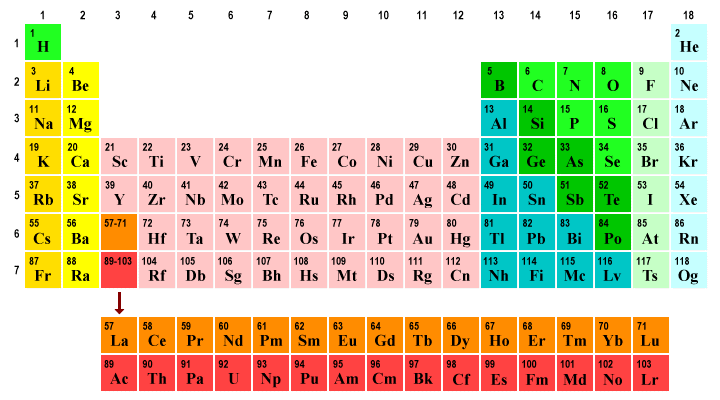The periodic table organises elements into periods (rows 1-7) and groups (columns 1-18)

More detailed versions of the periodic table (you can find an excellent example here) often show the electron configuration as a comma-separated list of values showing the number of electrons in each shell. For example, silicon (Si) would have the electron configuration 2, 8, 4. Electron shells 1n and 2n are full, containing two and eight electrons respectively, while electron shell 3n contains four electrons for a total of fourteen electrons.

We said earlier that electron shells in an atom are usually filled in strict order, starting with the lowest energy level. This is clearly the case for the elements in first three periods in the periodic table. After that, things get a little more complicated. In the fourth period, for example, we see electrons appearing in shell 4n before shell 3n is completely full. The reasons for this apparent anomaly will hopefully become clear in due course, when we start to look at orbitals.

The illustration below shows the first ten elements in the periodic table. Note that helium and neon both have full outer shells. Both of these elements can be found in Group 18 in the periodic table. All Group 18 elements (except for helium) have eight electrons in their valence shell. This makes them very stable and non-reactive. The Group 18 elements helium, neon, argon, krypton, xenon and radon, once known as the inert gases, are today more often referred to as the noble gases because they tend not to interact with other elements.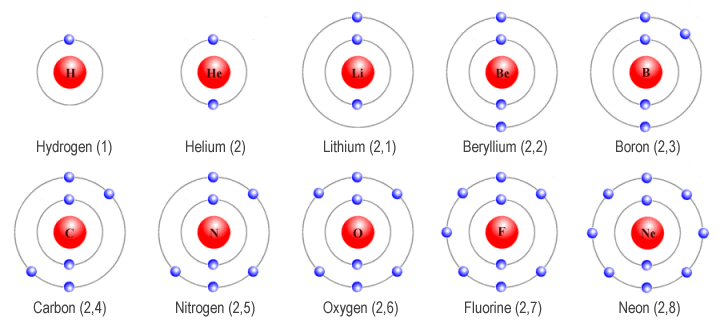The first ten elements in the periodic table

The electron configuration of an element, and in particular the number of electrons it has in its valence (outer) shell, has a huge influence on how atoms of that element interact with atoms of other elements. For example, the Group 17 elements fluorine, chlorine, bromine and iodine (collectively known as the halogens) all need one additional electron in order to achieve a full valence shell.

These elements react vigorously with the so-called alkali metals (lithium, sodium, potassium, rubidium and caesium) in Group 1 of the periodic table, all of which have just one electron in their valence shells. When sodium reacts with chlorine, these elements form the highly stable ionic compound sodium chloride (NaCl) - better known as common salt. By combining in this way, both elements effectively achieve complete outer shells.

## Orbitals

We know now of course that electrons do not move around the nucleus of an atom in the neat circular orbits described by the Rutherford-Bohr model. In fact, thanks to the work of Heisenberg and Schrödinger, we know that at any given time, an electron could be anywhere inside the electron cloud. Which is where orbitals come in.

We have stated that we cannot know with any certainty where an electron will be at any given time. We can, however, identify a three-dimensional region within the space occupied by the atom where a particular electron can be found for ninety percent (90%) of the time. We call this region an orbital. The name is rather misleading, since an electron orbital does not have anything to do with an "orbit".

Orbitals can have different shapes, and the geometry can get quite complex. The simplest kind of orbital is the s orbital, which is spherical in shape, with the nucleus of the atom at its centre. Other orbitals include the p, d and f orbitals. For historical reasons the designated letters stand for sharp, principal, diffuse, and fundamental, based on the spectral line observations associated with them, but the association is no longer of any significance. Orbits beyond the f orbitals are designated as g, h, i and so on (i.e. in alphabetical order).

The p orbital is shaped like a dumbell, again centred on the nucleus of the atom. Orbitals of this kind can co-exist within an electron shell, but each is aligned on a different perpendicular axis (imagine an x, y or z axis passing through the nucleus of the atom). It is not practical to try and describe the geometry of all of the different kinds of electron orbital, but the following graphic shows some of the possible permutations.Electron orbitals can have complex geometries (image: chem.libretexts.org)

Each of the orbitals shown in the illustration above, even the ones that look quite complex, can only contain a maximum of two electrons. The periodic table now contains more than a hundred elements, so you can probably imagine how complicated the orbital geometry can become! Fortunately, you are not usually required to describe anything too advanced in terms of orbital geometry in an exam. It is perhaps more important to understand how orbitals relate to electron shells.

Even though we know that electron shells do not actually consist of electrons travelling in neat circular orbits around the nucleus, they are real enough in the sense that each electron shell (sometimes called a principal energy level) represents a specific quantum energy level, represented by the principal quantum number (n). Each electron shell consists of one or more subshells, and each subshell consists of one or more orbitals.

It is often stated that electrons in the same electron shell have the same energy levels. This is at best a generalisation. All electrons in the same subshell will have exactly the same energy level. However, whist the overall energy levels in electron shells increases with the principal quantum number n, energy levels between subshells in the same electron shell will be different. Electrons in subshells that fill later will have more energy than those in subshells that fill earlier. In some cases, the energy ranges associated with two electron shells overlap, as we shall see.

Note that as well as being identified by the designated letter (s, p, d, f, etc.), orbitals can also be associated with a particular electron shell using the appropriate number. For example, the s orbitals in the first three electron shells will be refered to as the 1s, 2s and 3s orbitals. Likewise, the p orbitals in electron shells 2n, 3n and 4n will be referred to as the 2p, 3p and 4p orbitals respectively.

## Secondary, magnetic, and spin quantum numbers

Before we progress further, we would like to introduce some additional quantum numbers. The first of these is the secondary (or angular momentum, or azimuthal) quantum number, . This number identifies the shape of the orbital. We normally use the letters s, p, d, f, etc. for this purpose to avoid confusion with the principal quantum number (n). Values of relate to orbital types as follows:

Secondary quantum number ( ℓ)Orbital type
0s
1p
2d
3f
4g
5h
. . .. . .

The secondary quantum number identifies the subshell, since each subshell consists of the set of orbitals of a particular type within a shell. It also has significance with respect to the energy level of the subshell, which will increase slightly as increases.

The next quantum number we want to talk about is the magnetic quantum number, m. This number identifies the orientation of an orbital in space. All of the orbitals in a subshell will have the same energy values, but will have different spatial orientations. For any given subshell, the values that m can take will be integer values in the range - . . . 0 . . . . The number of orbitals in any given subshell will therefore be 2 + 1.

The last quantum number of interest in this context is the spin quantum number, s. This number gives the intrinsic angular momentum (or spin angular momentum or just spin) of an electron, and is designated by the letter s (the term intrinsic angular momentum simply means that the electron is spinning around its own axis).

For reasons that are beyond the scope of this page (and because a straightforward explanation has eluded even Nobel Prize winning physicists on occasion), the spin quantum number (s) of an electron can take values of s = + 1/2  or s = - 1/2 . These two spin orientations are sometimes referred to as spin-up and spin down, respectively. The important thing to note here is that, when two electrons occupy the same orbital, they must have opposite spin orientations.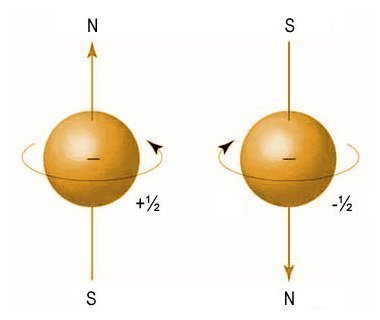An electron has a spin quantum number of - 1/2  or + 1/2

In 1925, the German physicist Friedrich Hermann Hund (1896-1997) established a set of rules governing electron configuration in atoms. The one that applies here (and the one commonly referred to as Hund's rule) is that, if two or more orbitals in the same subshell are available, electrons will occupy an empty orbital first, if one is available. Only after all of the orbitals in a subshell have one electron each will the electrons start to pair up. The first electron in an orbital has a spin of + 1/2 , and the second has a spin of - 1/2 .

The four quantum numbers (the principal quantum number n, the secondary quantum number , the magnetic quantum number m, and the spin quantum number s) together describe the unique quantum state of a single electron. They completely describe the movement and trajectories of each electron within an atom.

No two electrons can share the same combination of quantum numbers (a principle known as the Pauli Exclusion Principle, named after the Austrian physicist Wolfgang Ernst Pauli (1900-1958) who discovered it, and who made significant contributions in the field of spin theory (the study of the intrinsic angular momentum of elementary particles).

Quantum numbers are important for a number of reasons. They can be used to determine the electron configuration of an atom and the probable location of the electrons within an atom. They are also factors in determining characteristics such as the ionisation energy and atomic radius of an atom, how the atom will behave in a magnetic field, and whether or not the atom can generate its own magnetic field.

## Nodes

Most orbitals have one or more nodes. A node is a point at which the probablitity of an electron occurring is zero. There are two kinds of node - radial nodes, and angular nodes. A radial node is essentially the set of points that lie at some specified radial distance from the nucleus. An angular node is typically represented as a plane of symmetry bisecting the orbital.

With all types of orbital, the number of nodes increases with the principal quantum number (n). There are also some simple formulas that you can use if you need to determine the number and type of nodes for a particular orbital. These are as follows:

number of nodes  =  n - 1
number of angular nodes  =
number of radial nodes  =  n - - 1

where n is the principal quantum number and is the secondary quantum number. Let's look at a couple of examples to see how this works. Suppose we want to determine the number and type of the nodes in a p type orbital in the 3n electron shell. We know that the value of n is 3 (given), and we saw earlier that the value of for a p type orbital is 1. We therefore have:

number of nodes  =  n - 1  =  3 - 1  =  2
number of angular nodes  =    =  1
number of radial nodes  =  n - - 1  =  3 - 1 - 1  =  1

Now suppose that we want to find the number and type of the nodes in a d type orbital in the 5n electron shell. The numbers are getting bigger, but it's still a fairly trivial problem. The value of n is 5, and the value of for a d type orbital is 2. We therefore have:

number of nodes  =  n - 1  =  5 - 1  =  4
number of angular nodes  =    =  2
number of radial nodes  =  n - - 1  =  5 - 2 - 1  =  2

## The s orbitals

The simplest electronic configuration, unsurprisingly, can be seen in the hydrogen atom, which has just one electron. The hydrogen atom therefore has a single electron shell consisting of a single subshell containing a single s orbital. The s orbitals are spherical, and are the only orbitals that occur in every electron shell. Like all orbitals, the s orbitals have a number associated with them; the principal quantum number (n) of the shell in which they appear. The illustration below shows the first three s orbitals.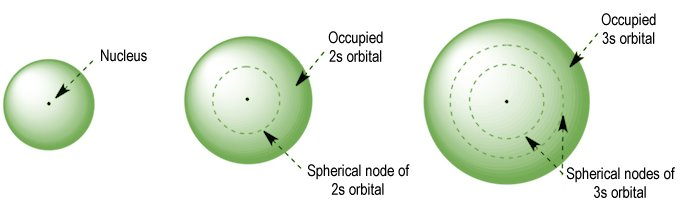The 1s, 2s and 3s orbitals

The s orbitals only have radial nodes (the 1s orbital has no nodes at all - it is simply a sphere of electron density). The following illustration should hopefully help you to visualise the electron distribution within the s orbitals.Electron distribution in the 1s, 2s and 3s orbitals

With all types of orbital - as we mentioned earlier - the number of nodes increases with the principal quantum number (n). The s orbitals are no exception - the 2s orbital has one radial node, the 3s orbital has two radial nodes, and so on (note that an electron shell may only contain one s orbital).

## The p orbitals

The 1n electron shell can contain only two electrons, both of which will occupy a single s orbital. The 2n electron shell, on the other hand, can contain up to eight electrons. Of these eight electrons, the first two will occupy the 2s orbital. Any additional electrons will occupy one of three p orbitals. The illustration below shows all of the orbitals found in the 1n and 2n electron shells.

As you can see, the three dumbbell-shaped p orbitals lie at right angles to one another along imaginary x, y and z axes. We identify individual p orbitals in an electron shell using the labels px , py , and pz .The 2n electron shell is the first to contain p orbitals

As we have seen, the value of the secondary quantum number () for p orbitals is one (1). This means that each p orbital has one angular node. You can imagine the angular node as a two-dimensional plane bisecting the nuclueus, with one lobe of the p orbital on either side of it. To give an example, the angular node for the px  orbital (that's the one that lies along the x axis) would be the plane on which both the y and z axes lie.

Since the probability of finding an electron at a node is zero, you might well be wondering how electrons manage to get from one lobe of a p orbital to the other. Whilst a detailed explanation is perhaps beyond the scope of this page, suffice it to say that it has to do with the fact that electrons can behave like both particles and waves.

Possible values for the magnetic quantum number m for a p orbital are -1, 0 and +1 (since is equal to one), which means that there can be three p orbitals in any of the electron shells except 1n. Once the s orbital in each electron shell has its complement of two electrons, the next six electrons will find a home in one of the p orbitals.

## The d orbitals

For the first eighteen elements in the periodic table up to and including argon (i.e. the first three periods of the table), all of the electrons will be found in either s orbitals or p orbitals. In fact, that continues to be the case for the first two elements of period four - potassium and calcium. In these two elements, we see electrons appearing in the s orbital of electron shell 4n, despite the fact that electron shell 3n is not yet full.

The reason for this seeming anomaly is that the energy level of the s orbital in electron shell 4n (designated as the 4s orbital) is actually slightly lower than the energy level of the d orbitals in electron shell 3n, and we have already seen that electrons will (usually) occupy the orbitals with the lowest energy levels first (just to make things more complicated, the energy level of the 4s orbital increases when the 3d orbitals start to fill up, but we're not going to get into that here).

The element scandium - one of the so-called transition metals - follows calcium in the periodic table. Scandium has nine (9) electrons in the 3n electron shell. Of these, two are accommodated in the 3s orbital and six more in the 3p orbitals. The ninth electron occupies a d orbital. This is where things start to get really interesting.

The d orbitals have a secondary quantum number () of two (2). Each d orbital therefore has two angular nodes, and possible values for the magnetic quantum number m of -2, -1, 0, +1 and +2, which means that there can be five d orbitals in any of the electron shells from 3n onwards. Most of the d orbitals look a bit like a four-leaf clover made from two pairs of dumbbells, although one looks like a dumbbell encircled by a doughnut! The illustration below should help you to visualise them.Electron shells 3n and upwards contain d orbitals

The five d orbitals in the 3n electron shell, together with the single s orbital and the three p orbitals, allow it to contain a total of eighteen (18) electrons (remember that each orbital can contain only two electrons, regardless of the complexity of its geometry).

As with the p orbitals, individual d orbitals are identified according to their relationship with arbitrary x, y and z axes. The dyz , dxz , and dxy  orbitals lie on the planes formed by the y and z, x and z, and x and y axes respectively, with each lobe forming a forty-five-degree angle with each axis on that plane. In the dx 2-y 2 orbital, one pair of lobes lies on the x axis and the other lies on the y axis.

The dz 2 orbital has a somewhat novel configuration, in which two lobes lie on the z axis and are encircled by a doughnut-shaped orbital centred on the z axis. The doughnut is bisected through its circumference by the plane formed by the x and y axes.

## The f orbitals

Electron shell 4n contains thirty-two (32) electrons. The first eighteen of these electrons occupy the s, p and d orbitals. The last fourteen electrons occupy f orbitals. In fact, the f orbitals do not begin to fill until we get to the third element in period five of the periodic table, yttrium (Y) - after both the 5p and the 6s orbital have been populated (because these orbitals have lower energy levels than the 4f orbitals).

The f orbitals have a secondary quantum number () of three (3). Each f orbital therefore has three angular nodes, and possible values for the magnetic quantum number m of -3, -2, -1, 0, +1, +2 and +3, which means that there can be seven f orbitals in any of the electron shells from 4n onwards.

The seven f orbitals in the 4n electron shell, together with the single s orbital, three p orbitals and four d orbitals, allow it to contain a total of thirty-two (32) electrons. The geometry of the f orbitals is more complex than any of the previous orbitals we have looked at. We will not attempt to describe them here, but the illustration below should help you to visualise them.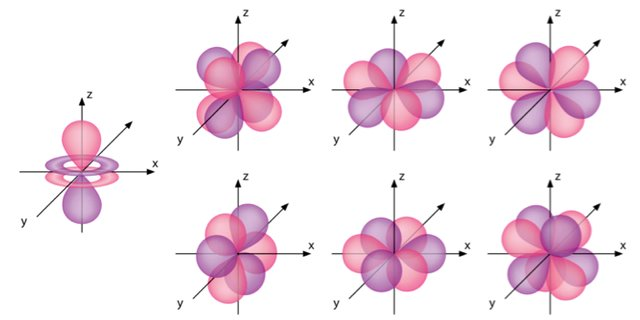Electron shells 4n and upwards contain f orbitals

The number of electron shells could in theory increase indefinitely, and we could expect to see electrons populating g, h and i orbitals (and beyond). However, although the periodic table contains elements with partially filled 5n, 6n and 7n electron shells, no naturally occurring or man-made element has ever been observed to have more than thirty-two electrons in any of its electron shells. From a practical viewpoint, therefore, our interest in orbitals ends with the f orbital.

## The aufbau principle

The aufbau principle takes its name from the German word Aufbauprinzip, which roughly translated means building-up principle. The principle, which dates from the 1920s, has been attributed to Niels Bohr and Wolfgang Pauli. It states the following:

"The orbitals of lower energy are filled in first with the electrons and only then the orbitals of high energy are filled."

The principle follows from the natural tendency for atoms to assume the most stable electron configuration possible. We have already seen that an electron shell does not need to be completely filled before the next electron shell starts to fill with electrons, because the energy levels of the suborbitals in two electron shells can overlap. So, are there any rules that we can apply here?

Actually, there is a relatively simple rule that can be used to determine - at least in the majority of cases - the order in which electrons fill orbitals. The German physicist Erwin Madelung (1881-1972) developed the Madelung rule (also known by several other names), which states that atomic orbitals are filled in order of increasing values of n + (i.e. the sum of the principal and secondary quantum numbers). The rule is often represented graphically using the following diagram: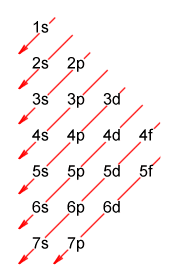A diagrammatic representation of the Madelung rule

Hopefully, the diagram is relatively easy to follow. To find the order in which the subshells fill with electrons, simply follow the arrows from top to bottom and from right to left. Each entry gives a principal quantum number (which identifies the electron shell) and a letter that represents one of the orbital types (s, p, d or f). Note that if two subshells have the same value of n + , the subshell with the lower value of n will fill first. Here is the complete sequence:

1s, 2s, 2p, 3s, 3p, 4s, 3d, 4p, 5s, 4d, 5p, 6s, 4f, 5d, 6p, 7s, 5f, 6d, 7p

Another way of representing this progression graphically is shown below. Each subshell is represented by a number of boxes corresponding to the number of orbitals it contains of a particular type. Electron shells are shown in a strict left-to-right order, but the sequence in which the subshells are populated is vertical (starting from the bottom of the diagram).This diagram shows the (approximate) order in which the electron subshells are filled

The aufbau principle, which was arrived at through extensive experimentation, is successful in predicting the electron configuration of all but a handful of elements (copper, chromium and palladium are examples of exceptions to the rule). Be aware, however, that it is not always so successful in predicting the precise order in which the subshells are filled, and anomalies do occur.

The element scandium (Sc) is a good example. Scandium has the atomic number twenty-one (21), and so has twenty-one electrons in its neutral (non-ionised) state. It is the first of the transition metals, and lies in period four of the periodic table. The Madelung rule successfully predicts the final electron configuration, which is as follows (note that the superscripted numbers represent the number of electrons in each orbital):

1s 2, 2s 2, 2p 6, 3s 2, 3p 6, 4s 2, 3d 1

Is this the order in which the subshells are populated, however? According to experimental results obtained so far, the answer is no. These experiments indicate that the single electron occupying the 3d orbital is present before the 4s orbital is filled.

A full examination of why this apparent anomaly occurs is beyond the scope of this page. Note, however, that it is important to be aware of such inconsistencies, especially when considering what happens during ionisation. In the case of scandium, a positive Sc+ ion is formed when an electron is lost from the 4s orbital. This makes sense, since the 4s orbital is the last orbital to be filled.

## Electron configuration shorthand

As we saw above with the element scandium, the electron configuration for any element can be written out using notation that tells us exactly what we need to know in terms of both the orbital type and the number of electrons in each subshell. For the lighter elements, this form of notation is relatively easy going. For example, the electron configuration for hydrogen is written as 1s 1; helium is written as 1s 2; and lithium is written as 1s 2, 2s 1.

As we can see from the scandium example, however, the longhand form of the notation becomes increasingly cumbersome as the atomic number increases. When we get to the heavier elements, it becomes clear that a shorthand version of the notation would certainly make life a little easier. Fortunately, an abbreviated form of the notation can be used.

The most frequently used shorthand version of electron configuration seems to be the noble gas configuration, so-called because it uses the noble gas occupying the last column of the periodic table in the row immediately preceding the element of interest. The notation essentially consists of the symbol for this noble gas, followed by the standard notation for the remaining subshell(s).

To find the noble gas configuration for an element, use the following steps:

1. Find the element you wish to find the configuration for in the periodic table.
2. Determine which noble gas to use (this will be the last element in the row above the element you wish to find the configuration for).
3. Write the symbol for the noble gas within square brackets, e.g. [He], [Ne], [Ar] etc., and make a note of both the atomic number of the noble gas and the row number in which it occurs.
4. Determine how many electrons remain (this will be the atomic number of the element you are interested in, minus the atomic number of the noble gas).
5. Begin the remainder of the configuration with the number of the row in which the element of interest resides, followed by orbital type s (we always start with orbital type s).
6. Continue writing your electron configuration according to the aufbau diagram we saw earlier until the remaining electrons have all been accounted for.

An example should help to clarify the procedure. Let's find the noble gas configuration for selenium (Se). The image below shows the relevant part of the periodic table.Part of the periodic table, with selenium and argon highlighted

Selenium is in row four of the periodic table, so the noble gas we must use is argon, which is in the last column of row three (3) and has the atomic number eighteen (18). We can now start our configuration by writing the symbol for argon in square brackets, thus:

[Ar]

The atomic number of selenium is thirty-four (34). Subtracting the atomic number of argon gives us a total of sixteen (16) electrons that still need to be accounted for. So far so good. We know that selenium is in row four, so we can write:

[Ar] 4s . . .

We still need to account for sixteen electrons. The 4s subshell accounts for two of them. Here is the sequence in which the subshells (from 4s onwards) are filled according to the aufbau principle:

4s, 3d, 4p, 5s, 4d, 5p, 6s, 4f, 5d, 6p, 7s, 5f, 6d, 7p

The complete 3d subshell will add ten (10) more electrons to the total, leaving just four electrons to find. Since the 4p subshell can hold a total of six electrons, we need look no further than that. Our final (abbreviated) configuration looks like this:

[Ar] 4s 2, 3d 10, 4p 4